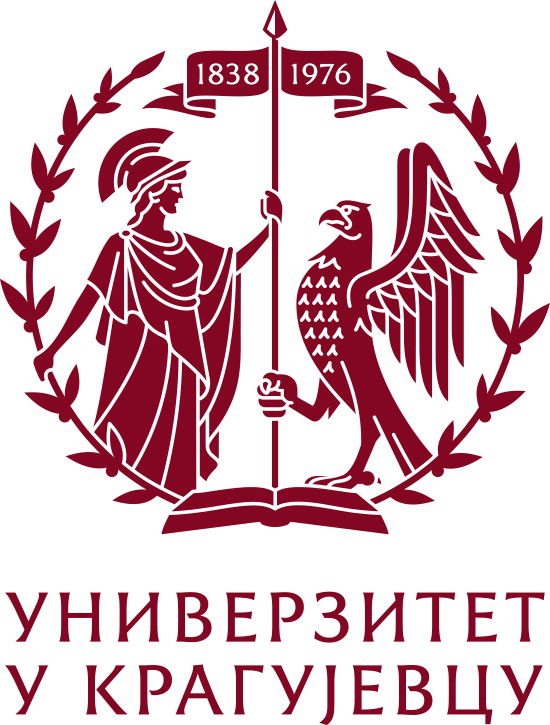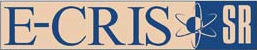Please use this identifier to cite or link to this item: `https://scidar.kg.ac.rs/handle/123456789/13901`
 Title: On bond incident degree indices of (n, m)-graphs Authors: Ali, AkbarGutman, IvanSaber H.Alanazi A. Journal: Match Issue Date: 1-Jan-2022 Abstract: A bond incident degree (BID) index of a graph G is defined as ∑ f(dG(u), dG(v)), with summation ranging over all pairs of adjacent vertices u, v of G, where dG(w) denotes the degree of the vertex w of G, and f is a real-valued symmetric function. This paper reports extremal results for BID indices of the type Ifi(G) = ∑ [fi(dG(u))/dG(u) + fi(dG(v))/dG(v)], where i ∈ {1, 2}, f1 is strictly convex, and f2 is strictly concave. Graphs attaining minimum If1 and maximum If2 are characterized from the class of connected (n, m)-graphs and chemical (n, m)-graphs, where n and m satisfy the conditions 3n ≥ 2m, n ≥ 4, m ≥ n + 1. By this, we extend and complement the recent result by Tomescu [ MATCH Commun. Math. Comput. Chem. 85 (2021) 285-294], and cover several well-known indices, including general zeroth-order Randić index, multiplicative first and second Zagreb indices, variable sum exdeg index, and Lanzhou index. URI: https://scidar.kg.ac.rs/handle/123456789/13901 Type: Article DOI: 10.46793/match.87-1.089A ISSN: 03406253 SCOPUS: 85116647895 Appears in Collections: Faculty of Science, Kragujevac
[ Google Scholar ]

54